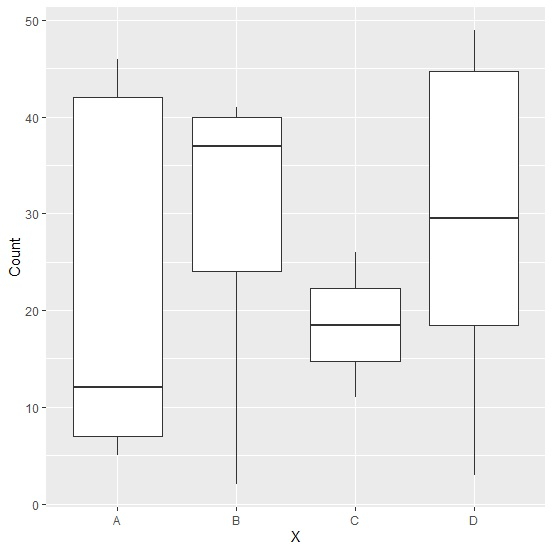# How to create a boxplot using ggplot2 with aes_string in R?

To create a boxplot using ggplot2 with aes_string in R, we can follow the below steps −

• First of all, create a data frame with one string and one numerical column.
• Then, use aes_string function of ggplot2 package to create the boxplot.

## Create the data frame

Let's create a data frame as shown below −

Live Demo

X<-sample(LETTERS[1:4],20,replace=TRUE)
Count<-sample(1:50,20)
df<-data.frame(X,Count)
df

On executing, the above script generates the below output(this output will vary on your system due to randomization) −

 X Count
1 A 12
2 B 41
3 A 5
4 A 42
5 C 26
6 D 14
7 D 20
8 B 37
9 A 46
10 D 31
11 A 7
12 D 28
13 B 40
14 D 47
15 D 49
16 D 3
17 C 11
18 D 44
19 B 24
20 B 2

## Create the boxplot

X<-sample(LETTERS[1:4],20,replace=TRUE)
ggplot(data=df,aes_string(x="X",y="Count"))+geom_boxplot()